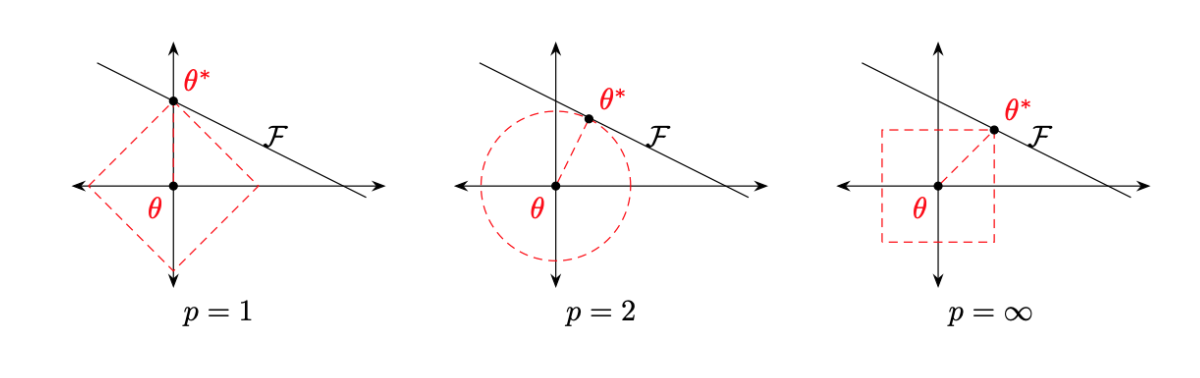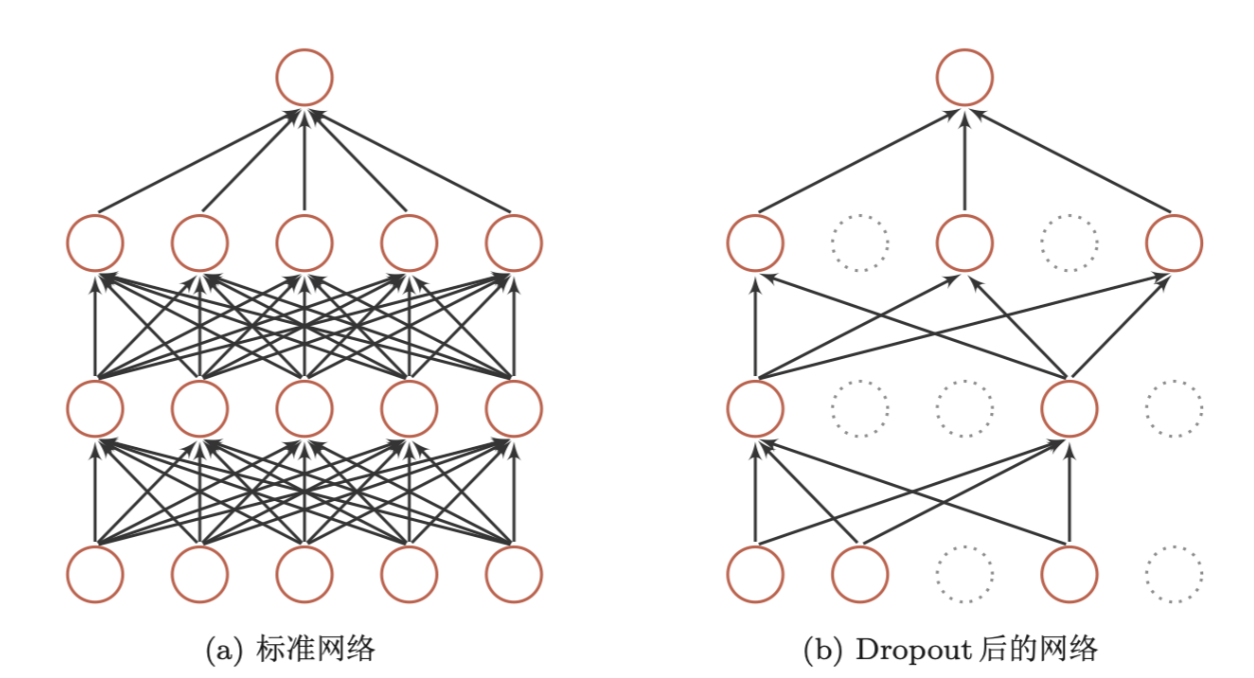• 第一篇包括
• 网络优化和正则化概述
• 优化算法介绍
• 第二篇包括
• 参数初始化
• 数据预处理
• 逐层归一化
• 第三篇包括
• 超参数优化
• 第四篇包括
• 网络正则化

### $l_1,l_2$正则

$l_1,l_2$正则是机器学习中常用的正则化方法，通过约束参数的$l_1,l_2$范数来减少模型在训练数据上的过拟合现象。

$L$为损失函数，$N$为训练的样本数量，$f(.)$为待学习的神经网络，$\theta$为参数，$l_p$为$l_1,l_2$正则中的一个，$\lambda$为正则项系数。### 丢弃法（1）集成学习的解释

（2）贝叶斯学习的解释

### 数据增强

• 转换（Rotation）：将图像按照顺时针或者逆时针方向随机旋转一定的角度；
• 翻转（Flip）：将图像沿水平或者垂直方向随机翻转一定的角度；
• 缩放（Zoom in/out）：将图像放大或者缩小一定的比例；
• 平移（Shift）：将图像按照水平或者垂直的方法平移一定步长；
• 加噪声（Noise）：加入随机噪声。